# Revisiting Nesterov’s Acceleration

Nesterov’s accelerated gradient descent (AGD) is hard to understand. Since Nesterov’s 1983 paper people have tried to explain “why” acceleration is possible, with the hope that the answer would go beyond the mysterious (but beautiful) algebraic manipulations of the original proof. This search for a good “why” has accelerated in the recent years under the impulsion of the machine learning community, as one would like to generalize Nesterov’s acceleration in various ways to deal with machine learning applications. Obviously generalizing is difficult when even the basic phenomenon is not well understood. Recent works in the “why” direction are re-interpreting the AGD algorithm from different point of views:

Allen-Zhu and Orrechia view AGD as a linear coupling of Gradient Descent and Mirror Descent. This gives a nice new proof where the acceleration results from a primal-dual point of view on the progress of the algorithm.

Su, Boyd and Candes (building on earlier work of O’Donoghue and Candes) view AGD as the discretization of a certain second-order ODE. Other papers in a somewhat similar direction include the work of Lessard, Recht and Packard; as well as Flammarion and Bach. Note that these papers do not give alternative proofs of acceleration for general smooth functions.

– Other relevant references include a recent paper of Arjevani, Shalev-Shwartz and Shamir, and a blog post by Hardt.

In joint work with Yin-Tat Lee and Mohit Singh we discovered a simple geometric reason for the possiblity of acceleration, which can be turned into a new algorithm with the same theoretical guarantees as AGD, and improved performances in practice. The proof of the convergence rate is 3 lines, assuming a completely elementary geometric fact (in dimension 2) which itself takes less than half a page to prove. The general principle is loosely inspired from the ellipsoid method (except that we work with balls, which makes the algorithm much more efficient). Let me guide you through this.

Preliminaries

Ok so we are trying to minimize on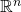a functionwhich is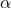-strongly convex and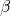smooth (see here for definitions). Denotethe minimizer of,the condition number of,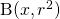for a Euclidean ball centered atand of radius squared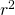,which are respectively a standard and long step of gradient descent. The crucial observation is that the local information atand the assumptions onallow to enclose the minimizer in some ball, precisely:Finally we need some fact on the intersection of balls. Take a ball with radius squared, and intersect it with some other ball which has the center of the previous ball on its boundary. This intersection is obviously contained in a ball of radius squared. An easy calculation shows that if the radius squared of one ball shrinks by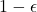then the intersection is included in a ball of radius squared. Furthermore if both balls shrink (additively) by the same amount (which is anfraction of the radius squared for one of the balls), then the intersection is included in a ball of radius squared. As we will see this fact is the reason why one can accelerate.

A suboptimal algorithm

Assume that we are given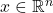such that. As we saw before one also hasTaking the intersection of these two balls and using the fact from the previous section we see that there existssuch thatThus by iterating the map fromto(defined implicitely above) we obtain a new algorithm with the same rate of convergence as gradient descent.

Acceleration

Now assume that the pointfrom the previous section and its guaranteeon the distance to the optimum came from the local information at some other point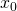, that isand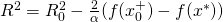where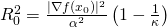. Assume furthermore thatis at least as good as a step of gradient descent at, that is. Then we can use the local information atto reduce the ball around, indeed one hasand thusFurthermore, as in the last section, one hasTaking the intersection of the above balls and enclosing it in another ball one obtains (see preliminaries) that there existssuch that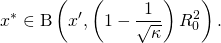The only issue at this point is that to iterate the map fromtoone would need thatis at least as good as a step of gradient descent on, which is not necessarily true (just as the assumptionis not necessarily true). We circumvent this difficulty by doing a line search on the line going throughand. The details (which are straightforward given the above discussion) are in the paper, specifically in Section 3. We also report encouraging preliminary experimental results.

This entry was posted in Optimization. Bookmark the permalink.

## 7 Responses to "Revisiting Nesterov’s Acceleration"

•••